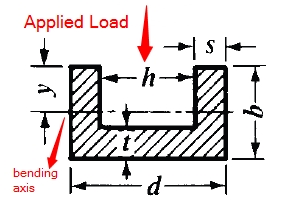This is the U Beam Moment of Inertia Calculator. Simply enter the dimensions below for U beam to find the moment of inertia.d = h = b = t = s =